#### Theory of Correlation and Pearson's Correlation Coefficient

Correlation:

Sum of Squares:

We introduced a notation prior in the course termed as the sum of squares. This notation was SS notation, and will make such formulas much simpler to work with.

SS(x) = Σx2 – (Σx)2/n
SS(y) = Σy2 – (Σy)2/n
SS(xy) = Σxy – (Σx)( Σy)/n

From above it can be noted that all are of same pattern.

SS(x) could be written as:

SS(x) = Σxx - (Σx)( Σx)/n

It is also noted that: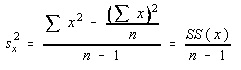Pearson's Correlation Coefficient:

There is a measurement of the linear correlation. The population parameter is symbolized by the greek letter rho and the sample statistic is symbolized by the roman letter r.

Some properties of r are as follows:

a) r just measures the strength of the linear relationship. There are other types of relationships besides linear.

b) r is always between -1 to 1 inclusive. -1 signifies perfect negative linear correlation and +1 signifies perfect positive linear correlation

c) r has similar sign as the slope of regression (best fit) line.

d) r doesn’t change if the independent (x) and dependent (y) variables are swapped

e) r doesn’t change when the scale on either variable is modified. You might multiply, divide, add or subtract a value to or from all x-values or y-values devoid of changing the value of r.

f) r consists a Student's t-distribution.

The formula for r is as shown below. Do not worry about it; we are not going to find it in this way. This formula can be simplified via some simple algebra and then some substitutions employing the SS notation.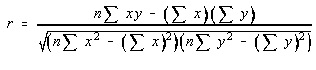Whenever you divide the numerator and denominator by n, then you get something that is starting to hopefully look recognizable. Each of such values has been seen prior to in the Sum of Squares notation section. Therefore, the linear correlation coefficient can be written in terms of sum of squares.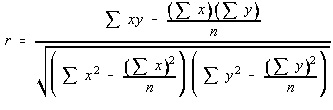This is the formula which we would be employing for computing the linear correlation coefficient when we were doing it by hand. Luckily for us, TI-82 has this computation built into it, and we do not have to do it by hand at all.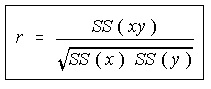Hypothesis Testing:

The claim we will be testing is ‘There is important linear correlation’.

The Greek letter for r is rho; therefore the parameter employed for the linear correlation is rho

H0: rho = 0
H1: rho <> 0

r consists of a t-distribution with n-2 degrees of freedom, and the test statistic is provided by:

t = r √(n-2)/(1-r2)

Now, there are n-2 degrees of freedom this time. This is the difference from prior to. Since an over-simplification, you subtract one degree of freedom for each and every variable, and as there are 2 variables then the degrees of freedom are n-2.

This does not appear like:

Test static = (Observed - Expected)/Standard error

Whenever you consider the standard error for r:

Standard error = √(1-r2)/(n-2)

The formula for test statistic is: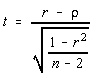Keep in mind that Hypothesis testing is always done beneath the supposition that the null hypothesis is true.

As H0 is rho = 0.

1-r2 is later recognized as the coefficient of non-determination.

Hypothesis Testing Revisited:

When you are testing to see if there is a significant linear correlation (that is, a two tailed test), then there is other way to execute the hypothesis testing. There is a table for critical values for Pearson's Product Moment Coefficient (or PPMC). The degree of freedom is (n-2).

Test statistic in this case is just the value of r. We can compare the absolute value of r to the critical value in the table. When the test statistic is more than the critical value, then there is important linear correlation. Moreover, you are able to state that there is significant positive linear correlation when the original value of r is positive and significant negative linear correlation when the original value of r was negative.

This is the most general method used. Though, the first method, with the t-value should be used if it is not a two-tail test, or when a distinct level of significance (that is, other than 0.01 or 0.05) is desired.

Causation: When there is a significant linear correlation among two variables, then one of five conditions can be true.

a) There is a direct effect and cause relationship.
b) There is a reverse effect and cause relationship.
c) The relationship might be caused by the third variable.
d) The relationship might be caused by the complex interactions of some variables.
e) The relationship might be coincidental.

Latest technology based Statistics Online Tutoring Assistance

Tutors, at the www.tutorsglobe.com, take pledge to provide full satisfaction and assurance in Statistics help via online tutoring. Students are getting 100% satisfaction by online tutors across the globe. Here you can get homework help for Statistics, project ideas and tutorials. We provide email based Statistics help. You can join us to ask queries 24x7 with live, experienced and qualified online tutors specialized in Statistics. Through Online Tutoring, you would be able to complete your homework or assignments at your home. Tutors at the TutorsGlobe are committed to provide the best quality online tutoring assistance for Statistics Homework help and assignment help services. They use their experience, as they have solved thousands of the Statistics assignments, which may help you to solve your complex issues of Statistics. TutorsGlobe assure for the best quality compliance to your homework. Compromise with quality is not in our dictionary. If we feel that we are not able to provide the homework help as per the deadline or given instruction by the student, we refund the money of the student without any delay.